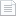검색어 입력폼

# [프로그래밍] 파일읽어최대최소구하기

등록일 2002.11.26텍스트 (txt) | 1페이지 | 가격 300원

없음

## 본문내용

#include<stdio.h>
#include<math.h>
void main(void)
{
double sum=0,avg;
double score;
double min,max;
int i=0,n;
FILE *fp;

fp=fopen("score.dat","r");

if(fp==NULL)
{
printf("\nFailed to open the file.\n");
}

while(fscanf(fp,"%lf",&score[i])!=EOF)
{
printf("score=%5.6lf\n",score[i]);
sum=sum+score[i];
i=i+1;

없음